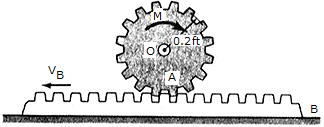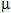Engineering Mechanics - PKRB: Impulse and Momentum - Discussion

Discussion :: PKRB: Impulse and Momentum - General Questions (Q.No.2)

2.Gear A has a weight of 1.5 lb, a radius of 0.2 ft, and a radius of gyration of ko = 0.13ft. The coefficient of friction between the gear rack B and the horizontal surface is= 0.3. If the rack has a weight of 0.8 lb and is initially sliding to the left with a velocity of (vB)2 = 8 ft/s to the left. Neglect friction between the rack and the gear and assume that the gear exerts only a horizontal force on the rack.

 [A]. M = 0.01425 lb-ft [B]. M = 0.00630 lb-ft [C]. M = 0.0622 lb-ft [D]. M = 0.0560 lb-ft

Explanation:

No answer description available for this question.